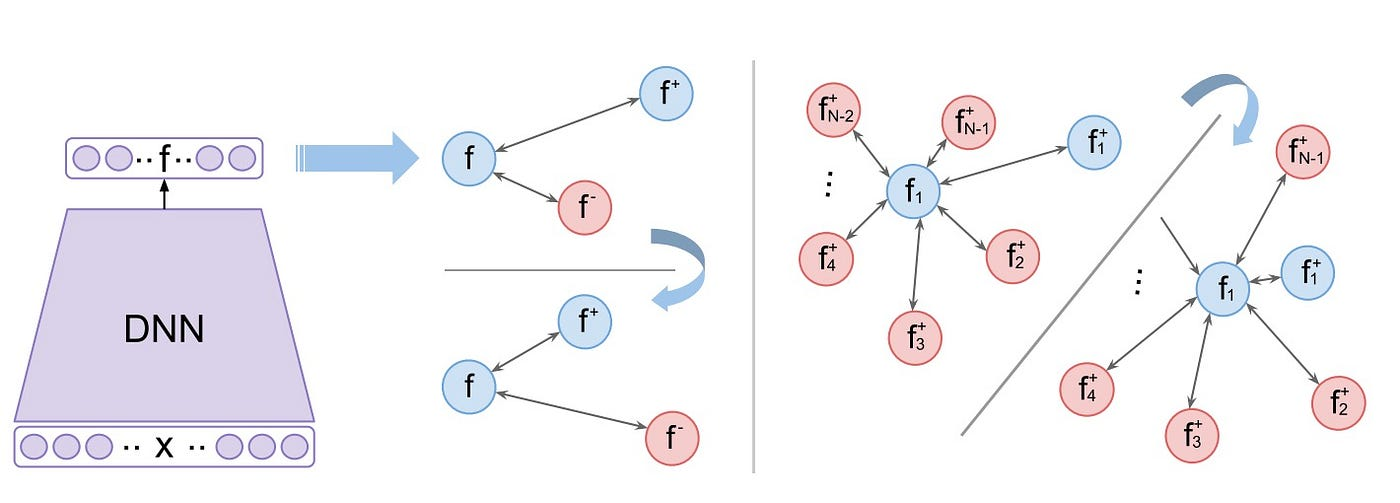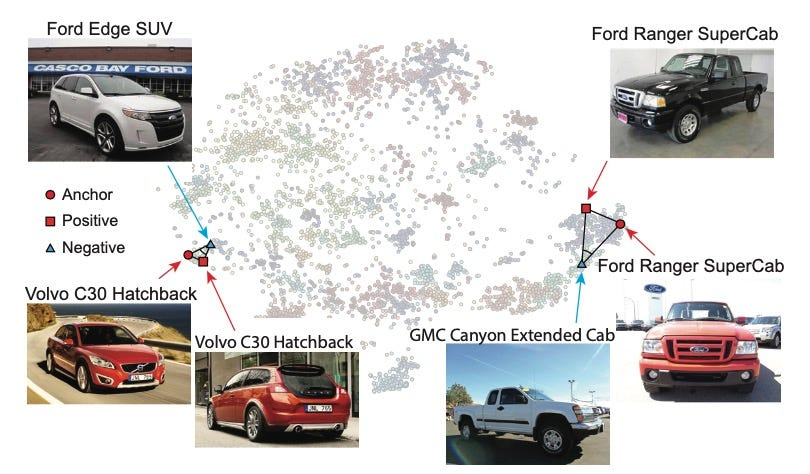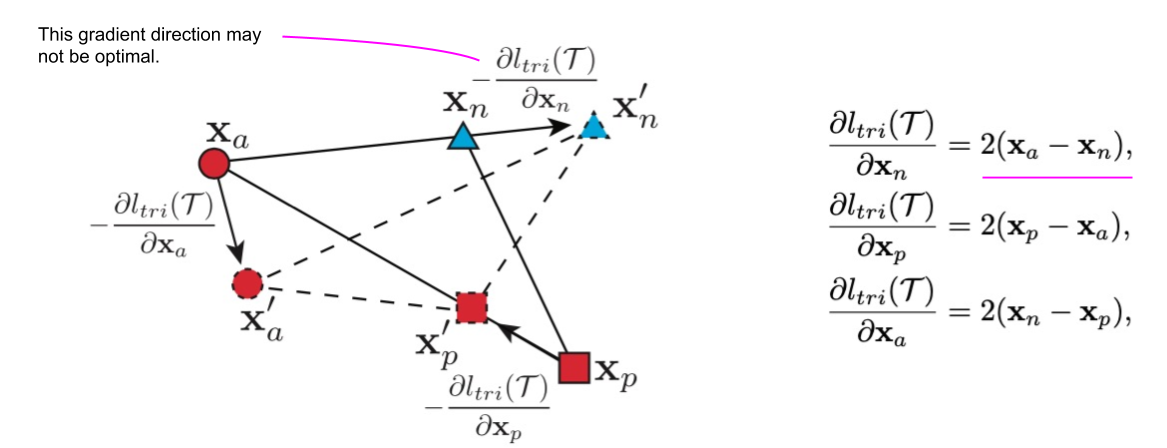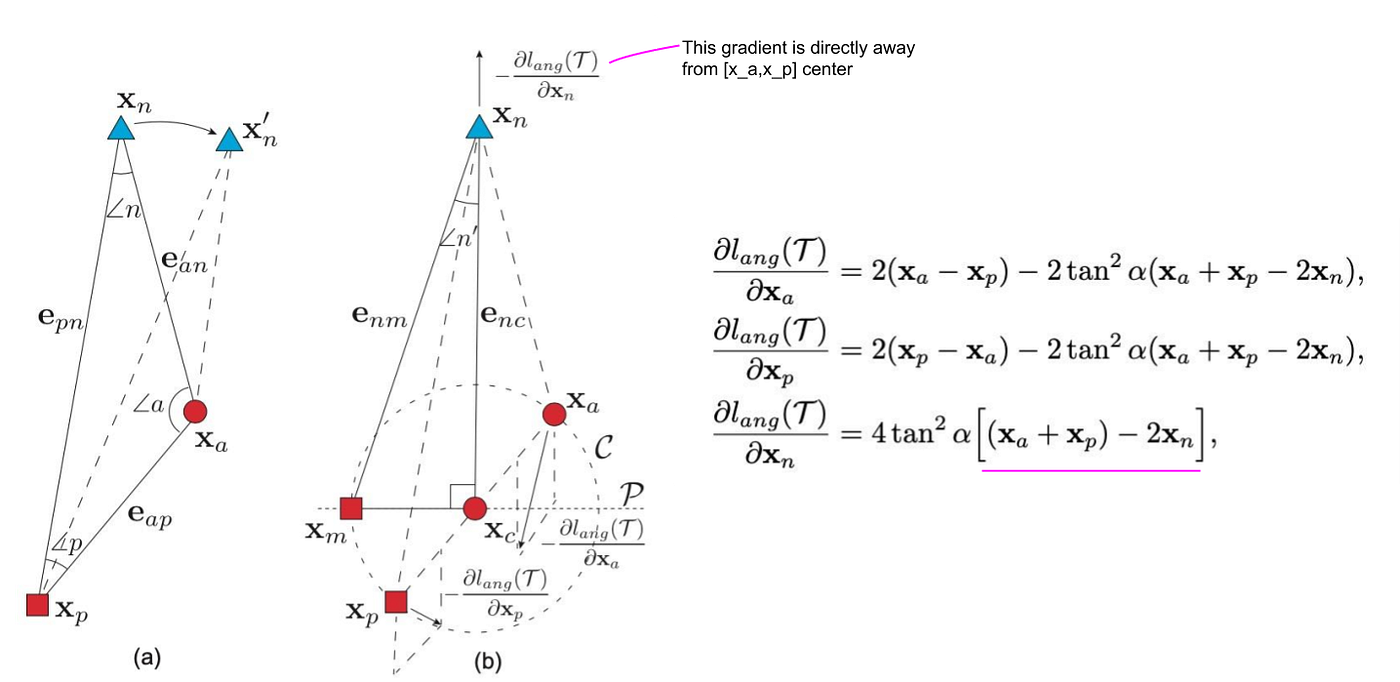# Retrieval with Deep Learning: A Ranking-Losses Survey Part 2N-pairs formulation using cosine similarity for a single triplet (a,p,n). The cosine similarity equals 1 for overlapping (identical) embeddings and 0 for opposite (different) embeddings.N-pairs formulation pairs every anchor f_a with a single positive f_p and every negative f_n in the mini-batch.(Left) Triplet loss pairs every anchor (f) with a single positive (f+) and negative (f-) sample. (Right) N-pairs pairs the anchor with a single positive (f+) and all negatives in the mini-batch.Example of feature embedding computed by t-SNE for the Stanford car dataset, where the images of Ford Ranger SuperCab (right) have a more diverse distribution than Volvo C30 Hatchback (left). Conventional triplet loss has difficulty in dealing with such an unbalanced intra-class variation. The proposed angular loss addresses this issue by minimizing the scale-invariant angle at the negative point.The negative point x_n gradient may not be optimal without the guarantee of moving away from the class which both the anchor x_a and positive sample x_p belong to; it is not perpendicular to line [x_a,x_p].Illustration of the angular constraint using a synthetic triplet. (a) Directly minimizing ∠n is unstable as it would drag x_n closer to x_a. (b) The more stable is minimizing ∠n′ defined by re-constructing the triangle [x_m,x_n,x_c] where x_c is the center between x_a,x_p.
• The sampling strategy used to train triplet loss can lead to significant performance differences. Hard Mining is efficient and converges faster if a model collapse is avoided.
• The nature of the training dataset is another important factor. When working with person re-identification or face clustering, we can assume that each class is represented by a single cluster, i.e., a single-mode with small intra-class variations. Yet, some retrieval datasets like CUB-200–2011 and Stanford Online Products have a lot of intra-class variations. Empirically, hard triplet loss works better for person/face re-identification tasks while N-pairs and Angular losses work better on CUB-200 and Stanford Online Product datasets.
• When approaching a new retrieval task and tuning the hyper-parameters (learning rate and batch_size) for a new training dataset, I found semi-hard triplet loss to be the most stable. It does not achieve the best performance but it is the least likely to degenerate.

--

--